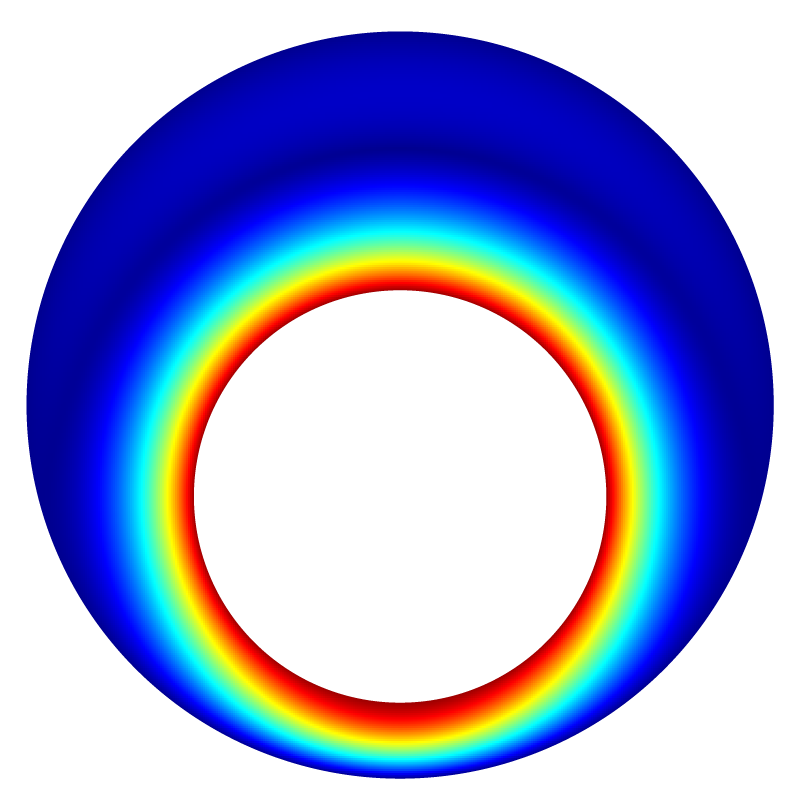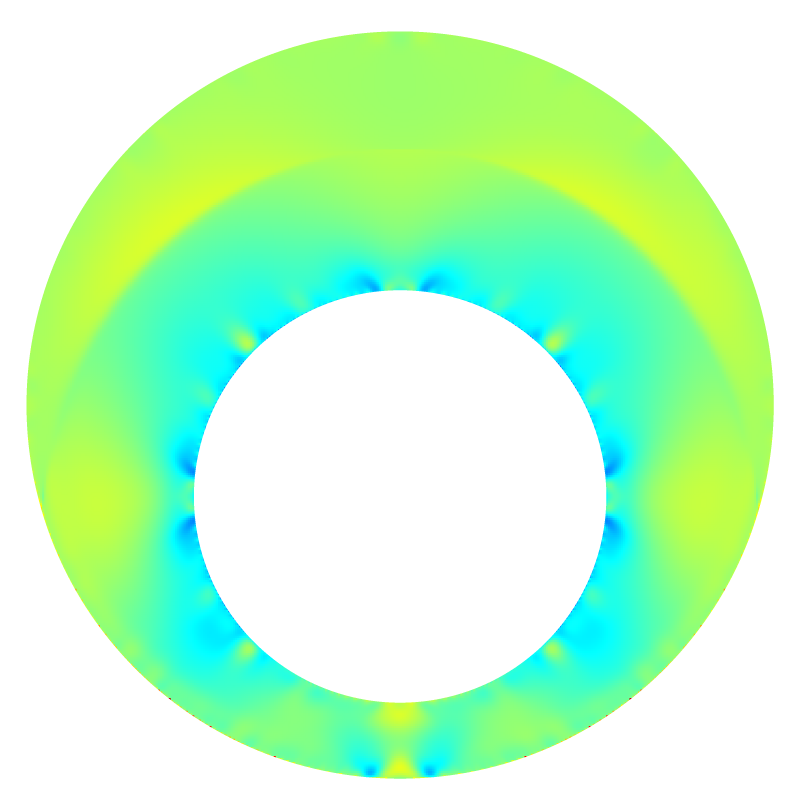# Wannier flow between rotating excentric cylinders

This test case is similar to Couette flow but with eccentric cylinders. While the concentric cylinders case can be reduced to a one-dimensional equation in polar coordinates (the radial velocity component vanishes), this is not the case for eccentric cylinders. For this problem (also known as “journal bearing” flow), an exact analytical solution in the limit of Stokes flows was obtained by Wannier, 1950 using conformal mapping.

#include "grid/multigrid.h"
#include "embed.h"
#include "navier-stokes/centered.h"
#include "view.h"

The analytical solution, as computed by Wannier.

void psiuv (double x, double y,
double r1, double r2, double e,
double v1, double v2,
double * ux, double * uy, double * psi)
{
double d1 = (r2*r2 - r1*r1)/(2.*e) - e/2.;
double d2 = d1 + e;
double s = sqrt((r2 - r1 - e)*(r2 - r1 + e)*(r2 + r1 + e)*(r2 + r1 - e))
/(2.*e);
double l1 = log((d1 + s)/(d1 - s));
double l2 = log((d2 + s)/(d2 - s));
double den = (r2*r2 + r1*r1)*(l1 - l2) - 4.*s*e;
double curlb = 2.*(d2*d2 - d1*d1)*(r1*v1 + r2*v2)/((r2*r2 + r1*r1)*den) +
r1*r1*r2*r2*(v1/r1 - v2/r2)/(s*(r1*r1 + r2*r2)*(d2 - d1));
double A = -0.5*(d1*d2-s*s)*curlb;
double B = (d1 + s)*(d2 + s)*curlb;
double C = (d1 - s)*(d2 - s)*curlb;
double D = (d1*l2 - d2*l1)*(r1*v1 + r2*v2)/den
- 2.*s*((r2*r2 - r1*r1)/(r2*r2 + r1*r1))*(r1*v1 + r2*v2)/den
- r1*r1*r2*r2*(v1/r1 - v2/r2)/((r1*r1 + r2*r2)*e);
double E = 0.5*(l1 - l2)*(r1*v1 + r2*v2)/den;
double F = e*(r1*v1 + r2*v2)/den;

y += d2;
double spy = s + y;
double smy = s - y;
double zp = x*x + spy*spy;
double zm = x*x + smy*smy;
double l = log(zp/zm);
double zr = 2.*(spy/zp + smy/zm);

*psi = A*l + B*y*spy/zp + C*y*smy/zm + D*y + E*(x*x + y*y + s*s) + F*y*l;
*ux = -A*zr - B*((s + 2.*y)*zp - 2.*spy*spy*y)/(zp*zp) -
C*((s - 2.*y)*zm + 2.*smy*smy*y)/(zm*zm) - D -
E*2.*y - F*(l + y*zr);
*uy = -A*8.*s*x*y/(zp*zm) - B*2.*x*y*spy/(zp*zp) -
C*2.*x*y*smy/(zm*zm) + E*2.*x -
F*8.*s*x*y*y/(zp*zm);
}

The strange choices for radii R1,R2 and eccentricity ECC come from the ‘bipolar’ variant.

#define R1 (1./sinh(1.5))
#define R2 (1./sinh(1.))
#define X1 (1./tanh(1.5))
#define X2 (1./tanh(1.))
#define ECC (X2 - X1)

int main() {

Space and time are dimensionless.

  size (2.5);
DT = HUGE;

origin (-L0/2., -L0/2.);

stokes = true;
for (N = 32; N <= 512; N *= 2)
run();
}

scalar un[];

#define WIDTH 0.5

event init (t = 0) {

Viscosity is unity.

  mu = fm;

The geometry is two excentric cylinders.

  solid (cs, fs, difference (sq(R2) - sq(x) - sq(y - ECC),
sq(R1) - sq(x) - sq(y)));

The outer cylinder is fixed and the inner cylinder is rotating with a tangential velocity unity.

  u.n[embed] = dirichlet (x*x + y*y > 1.5*sq(R1) ? 0. : - y/R1);
u.t[embed] = dirichlet (x*x + y*y > 1.5*sq(R1) ? 0. :   x/R1);

We initialize the reference field.

  foreach()
un[] = u.y[];
}

We look for a stationary solution.

event logfile (t += 0.01; i <= 1000) {
double du = change (u.y, un);
if (i > 0 && du < 1e-5)
return 1; /* stop */
}

We compute the error field and error norms and display the tangential velocity, pressure and error fields using bview.

event profile (t = end)
{
scalar e[], nu[];
foreach() {
if (cs[] > 0.) {
double pw, uw, vw;
psiuv (x, y - ECC, R1, R2, ECC, 1., 0., &uw, &vw, &pw);
nu[] = sqrt (sq(u.x[]) + sq(u.y[]));
e[] = nu[] - sqrt(uw*uw + vw*vw);
}
else
e[] = nu[] = p[] = nodata;
}

norm n = normf (e);
fprintf (stderr, "%d %.3g %.3g %.3g %d %d %d %d %d\n", N, n.avg, n.rms, n.max,
i, mgp.i, mgp.nrelax, mgu.i, mgu.nrelax);
dump();

view (fov = 13.85, tx = 0, ty = -0.088);

draw_vof ("cs", "fs", filled = -1, fc = {1,1,1});
save ("nu.png");

draw_vof ("cs", "fs", filled = -1, fc = {1,1,1});
save ("p.png");

draw_vof ("cs", "fs", filled = -1, fc = {1,1,1});
save ("e.png");

if (N == 32)
foreach() {
double pw, uw, vw;
psiuv (x, y, R1, R2, ECC, 1., 0., &uw, &vw, &pw);
fprintf (stdout, "%g %g %g %g %g %g %g %g\n",
x, y, u.x[], u.y[], p[], e[], uw, vw);
}
}

## ResultsNorm of the velocity

The pressure field is not trivial.Pressure fieldError field

Convergence is close to second-order.

set xrange [*:*]
ftitle(a,b) = sprintf("%.3f/x^{%4.2f}", exp(a), -b)
f(x) = a + b*x
fit f(x) 'log' u (log($1)):(log($4)) via a,b
f2(x) = a2 + b2*x
fit f2(x) '' u (log($1)):(log($2)) via a2,b2
set xlabel 'Resolution'
set logscale
set xtics 16,2,1024
set ytics format "% .0e"
set grid ytics
set cbrange [1:2]
set xrange [16:1024]
set ylabel 'Error'
set yrange [*:*]
set key top right
plot '' u 1:4 pt 6 t 'max', exp(f(log(x))) t ftitle(a,b), \
'' u 1:2 t 'avg', exp(f2(log(x))) t ftitle(a2,b2)Error convergence (script)

 [wannier1950] Gregory H. Wannier. A contribution to the hydrodynamics of lubrication. Quarterly of Applied Mathematics, 8(1):1–32, 1950. [ http ]